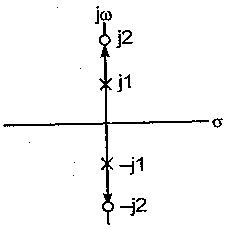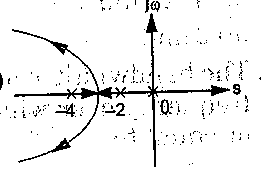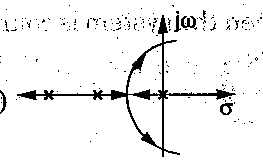# In the root-locus Fr open-loop transfer function  G(s)H(s) =K(s+6)/(s+3)(s+5).The 'break away' and 'break-in',  are located respectively at

1.  -2 and -1

2.  -2.47 and -3.77

3.  -4.27 and -7.23

4.  -7.73 and -4.27

4

-7.73 and -4.27

Explanation :
No Explanation available for this question

# The forward-path open-loop transfer function of a unity feedback system is G(s)=K(s2+4)/(s2+1) For this system root locus is

1.

2.

3.

4.

4Explanation :
No Explanation available for this question

# If open-loop transfer function of the system  G (s) H (s) =K(s + 10)/s(s + 8) (s + 16) (s + 72) then a closed loop pole will be locate all s =-12, when the value of K is

1.  4355

2.  5760

3.  9600

4.  9862

4

5760

Explanation :
No Explanation available for this question

# The root-locus plot of the open-loop transfer function G (s)H (s)=K/s s(s + 2) (s + 4) is

1.

2.

3.

4.

4Explanation :
No Explanation available for this question

# root locus plot of the system having the lot transfer function,G(s) H(s) =K/s(s + 4) (s2 + 4s + 5) has

1.  no breakaway point

2.  three real breakaway point

3.  only one breakaway point

4.  one real and two complex breakaway point

4

three real breakaway point

Explanation :
No Explanation available for this question

# An open loop transfer function is given by, s G(s) H(s) = K(s+1/)s(s + 2) (s2+ 1) + 25 + 2). It has

1.  one zero at infinity

2.  two zeros at infinity

3.  three zeros at infinity

4.  four zeros at infinity

4

three zeros at infinity

Explanation :
No Explanation available for this question

# The open-loop transfer function of a feedback control system is given by G(s) H(s)=K(s + 2)/s(s+ 4) (s2 + 4s + 8) In the root locus diagram of the system, the asymptotes of the root loci for large values of K meet a point in the s-plane. Which one of the following is the set of co-ordinate of that point

1.  (-1, 0)

2.  (-2, 0)

3.  (-10/3, 0)

4.  (2, 0)

4

(-2, 0)

Explanation :
No Explanation available for this question

# If characteristic equation of a closed-loop system is 1 +  K/s(s +1) (s +2)=0 then centroid at the asymptote's in root-locus will be

1.  zero

2.  2

3.  -1

4.  -2

4

-1

Explanation :
No Explanation available for this question

# The open loop transfer of a unity feedback control system is given by G(s)=K(s+2)/s(s2+2s+2) The centroid and angle of root locus asymptotes are respectively

1.  zero and +900,-900

2.  -2/3 and+600,-600

3.  zero and +1200,-1200

4.  -2/3 and -900,+900

4

zero and +900,-900

Explanation :
No Explanation available for this question

# Given a unity feedback system with open-loop transfer function G(s)=K/s(s+1)(s+2) The root locus plot of the system is of the form

1.

2.

3.

4.

4